##### Quantum Physics For Dummies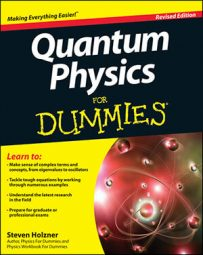Although Max Planck and Albert Einstein postulated that light could behave as both a wave and a particle, it was Arthur Compton who finally proved that this was possible. His experiment involved scattering photons off electrons, as the figure below shows, and offered proof for what we now refer to as the Compton effect.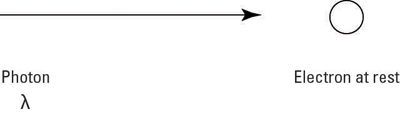Light incident on an electron at rest.

Incident light comes in with a wavelength of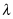and hits the electron at rest. After that happens, the light is scattered, as you see here: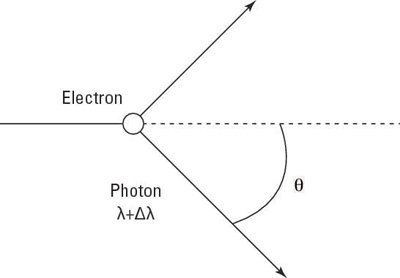Photon scattering off an electron.

Classically, here’s what should’ve happened: The electron should’ve absorbed the incident light, oscillated, and emitted it — with the same wavelength but with an intensity depending on the intensity of the incident light. But that’s not what happened — in fact, the wavelength of the light is actually changed by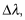called the wavelength shift. The scattered light has a wavelength of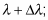in other words, its wavelength has increased, which means the light has lost energy. And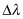depends on the scattering angle,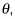not on the intensity of the incident light.

Compton could explain the results of his experiment only by making the assumption that he was actually dealing with two particles — a photon and an electron. That is, he treated light as a discrete particle, not a wave. And he made the assumption that the photon and the electron collided elastically — that is, that both total energy and momentum were conserved.

Making the assumption that both the light and the electron were particles, Compton then derived this formula for the wavelength shift (it’s an easy calculation) if you assume that the light is represented by a photon with energy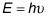and that its momentum is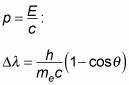where h is Planck’s constant, me is the mass of an electron, c is the speed of light, and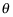is the scattering angle of the light.

You also see this equation in the equivalent form: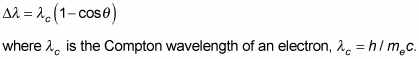And experiment confirms this relation — both equations.

Note that to derive the wavelength shift, Compton had to make the assumption that here, light was acting as a particle, not as a wave. That is, the particle nature of light was the aspect of the light that was predominant.